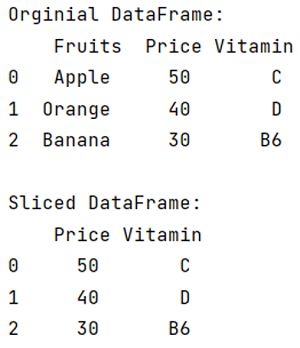# How to take column slices of DataFrame in pandas?

Given a DataFrame, we have to take column slice.
Submitted by Pranit Sharma, on May 15, 2022

Columns are the different fields that contain their particular values when we create a DataFrame. We can perform certain operations on both rows & column values. DataFrames are 2-dimensional data structures in pandas. DataFrames consists of rows, columns, and the data. DataFrame can be created with the help of python dictionaries or lists but in the real world, CSV files are imported and then converted into DataFrames. Sometimes, DataFrames are first written into CSV files.

In this article, we are going to learn how to slice the DataFrame column-wise, for this purpose we will use pandas.DataFrame.loc property.

## pandas.DataFrame.loc Property

This is a type of data selection method which takes the name of a row or column as a parameter. We can perform various operations using pandas.DataFrame.loc property. Inside loc property, the index value of the row comes first followed by the number of columns.

Syntax:

```DataFrame.loc
```

To work with pandas, we need to import pandas package first, below is the syntax:

```import pandas as pd
```

Let us understand with the help of an example:

```# Importing pandas package
import pandas as pd

# Creating dictionary
d = {
'Fruits':['Apple','Orange','Banana'],
'Price':[50,40,30],
'Vitamin':['C','D','B6']
}

# Creating DataFrame
df = pd.DataFrame(d)

# Display original DataFrame
print("Original DataFrame:\n",df,"\n")

# Slicing DataFrame column wise
result = df.loc[:,'Price':'Vitamin']

# Display result
print("Sliced DataFrame:\n",result)
```

Output:Top MCQs